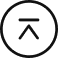回到顶部# 使用行空板制作屏幕取色卡 中等

HonestQiao 2022.11.17 398 9

``````img = Image.open("imgs/color_chart.png")
color = img.getpixel(100, 100)``````

``````img = Image.open("imgs/color_chart.png")
img2 = img.resize((240,240))
img.close()``````

``````# 控件的onclick触发
info_text = gui.draw_text(x=120, y=320, text='你好',origin='bottom' ,onclick=info_text_on_click)

# 鼠标的移动触发
def mouse_move(x, y):
info_text.config(text="坐标:x={},y={}".format(x, y))
print(x,y)

gui.on_mouse_move(mouse_move)``````

onclick触发调用的时候，只能知道有一次屏幕点击控件发生了，可以知道是在哪个控件上触发的，但是，并不知道具体点击的坐标位置。

on_mouse_move就可以。当在屏幕上触摸移动的时候，类似于在屏幕上移动鼠标，会实时的调用on_mouse_move对应的回调函数，并将当前坐标位置(x,y)做为参数传入。

``````# -*- coding: UTF-8 -*-
import sys
import time
from unihiker import GUI
from PIL import Image

# 屏幕鼠标移动回调
def mouse_move(x, y):
global move_time, mouse_status, color_chart_image, mouse_color

# 显示坐标值
pos_text.config(x=0,text="坐标:({},{})".format(x, y))

# 判断是否在色卡图片上移动
if y>=30 and y < 30+240:
# 在色卡图片中取色
mouse_color = color_chart_image.getpixel((x, y-30))
else:
# 超出则使用(0,0,0)
mouse_color = (0,0,0)

# 显示颜色之
color_text.config(x=100,text="颜色:({},{},{})".format(mouse_color,mouse_color,mouse_color))

# 更新预览图片颜色
color_img.config(color=mouse_color)

# 更新当前移动时间
move_time = time.time()

# 设置移动状态
mouse_status = 1

# 打开色卡图片
color_chart_image_default = Image.open("imgs/color_chart.png")
# 调整色卡图片大小
color_chart_image = color_chart_image_default.resize((240,240)).convert('RGB')
# 关闭默认打开的色卡图片
color_chart_image_default.close()

# 初始化屏幕
gui = GUI()
gui.clear()

# 初始化屏幕标识文本
gui.draw_text(x=50, y=0, text="WS2812B色卡", font_size=13)
gui.draw_image(x=0,y=30,w=240,h=240,image=color_chart_image)
pos_text = gui.draw_text(x=40, y=300, text='鼠标坐标', font_size=10)
color_text = gui.draw_text(x=150, y=300, text='颜色', font_size=10)

# 初始化颜色预览色块
color_img = gui.fill_round_rect(x=224, y=304, w=14, h=14, r=2, color=(255,255,255))

# 设定中间量初始值
move_time = 0
mouse_status = 0
mouse_color = (0,0,0)

# 屏幕鼠标移动回调绑定
gui.on_mouse_move(mouse_move)

# 循环
while True:
time.sleep(1)
``````他的勋章
展开更多

你可能感兴趣的